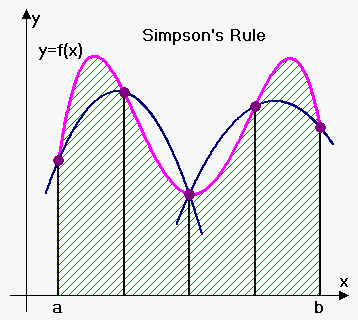# Simpson's Rule

## Related calculator: Simpson's Rule Calculator for a Function

Idea of Simpson's rule is in following: approximate curve by parabola and then find area of parabola (it is easy to do because we know antiderivative of quadratic function).

Again we divide [a,b] into n subintervals of equal length Delta x=(b-a)/n, and also require n to be even number.Then on each consecutive pair of intervals we approximate the curve y=f(x) by a parabola. If y_i=f(x_i), then P_i=(x_i,y_i) is the point on the curve lying above x_i.

A typical parabola passes through three consecutive points P_i, P_(i+1) and P_(i+2).

First we find equation of parabola that passes through points (x_0,y_0), (x_1,y_1) and (x_2,y_2).

Also note that x_1=x_0+Delta x and x_2=x_0+2Delta x.

Equation of any parabola has form y=Ax^2+Bx+C and so area under parabola from x=x_0 to x=x_2=x_0+2Delta x is

S=int_(x_0)^(x_0+2Delta x) (Ax^2+Bx+C)dx=(A/3 x^3+B/2 x^2+Cx)|_(x_0)^(x_0+2Delta x)=

=(A/3 (x_0+2Delta x)^3+B/2 (x_0+2Delta x)^2+C(x_0+2Delta x))-(A/3 x_0^3+B/2 x_0^2+Cx_0)=

=2ADelta x x_0^2+4A(Delta x)^2x_0+8/3A(Delta x)^3+2B Delta x x_0+2B(Delta x)^2+2CDelta x=

=(Delta x)/3(A(6x_0^2+12Delta x x_0+8A(Delta x)^2)+B(6x_0+6Delta x)+6C).

Additionally parabola should pass through points P_0=(x_0,y_0), P_1=(x_1,y_1) and P_2=(x_2,y_2) (recall that x_1=x_0+Delta x and x_2=x_0+2Deltax), so

{(y_0=Ax_0^2+Bx_0+C),(y_1=A(x_0+Delta x)^2+B(x_0+Delta x)+C),(y_2=A(x_0+2Delta x)^2+B(x_0+2Delta x)+C):}

From this we have that

y_0+4y_1+y_2=

=Ax_0^2+Bx_0+C+4(A(x_0+Delta x)^2+B(x_0+Delta x)+C)+A(x_0+2Delta x)^2+B(x_0+2Delta x)+C=

=A(6x_0^2+12Deltax x_0+8(Delta x)^2)+B(6x_0+6Delta x)+6C.

If we know multiply both sides of equality by (Delta x)/3 we will obtain that

(Delta x)/3 (y_0+4y_1+y_2)=(Delta x)/3(A(6x_0^2+12Delta x x_0+8(Delta x)^2)+B(6x_0+6Delta x)+6C).

But right side is exactly area S under parabola.

Therefore, S=(Delta x)/3(y_0+4y_1+y_2).

Similarly, it can be shown that the area under parabola through P_2, P_3 and P_4 from x=x_2 to x=x_4 is (Delta x)/3(y_2+4y_3+y_4).

In general, area under parabola through P_i, P_(i+1), P_(i+2) from x=x_i to x=x_(i+2) is (Delta x)/3(y_i+y_(i+1)+y_(i+2)).

Summing areas under parabolas on each subinterval we will obtain that int_a^bf(x)dx~~(Delta x)/3(y_0+4y_1+y_2)+(Delta x)/3(y_2+4y_3+y_4)+...+(Delta x)/3(y_(n-2)+4y_(n-1)+y_n).

Simpson's Rule. int_a^bf(x)dx~~S_n=(Delta x)/3(y_0+4y_1+2y_2+4y_3+2y_4+...+2y_(n-2)+4y_(n-1)+y_n).

where n is even and Delta x=(b-a)/n.

Note the pattern of coeffcients: 1,4,2,4,2,4,2,4,2,...,4,2,4,2,4,2,4,1.

Example 1. Use Simpson's Rule to approximate value of int_1^2 1/x^2dx with n=8.

Here  a=1, b=2, f(x)=1/x^2 and n=8. So, Delta x=(b-a)/n=(2-1)/8=0.125.

So, int_1^2 1/x(dx)~~S_n=

=(0.125)/3(f(1)+4f(1.125)+2f(1.25)+4f(1.375)+2f(1.5)+4f(1.625)+2f(1.75)+4f(1.875)+f(2))=

=0.125/3(1/1^2+4/(1.125)^2+2/(1.25)^2+4/(1.375)^2+2/(1.5)^2+4/(1.625)^2+2/(1.75)^2+4/(1.875)^2+1/2^2)~~0.5000299.

True value of integral is I=int_1^2 1/x^2dx=0.5. As can be seen Simpson's rule gave very good approximation.

When we approximate integral we will always have some error: E=int_a^bf(x)dx-App where App is approximation and E is error.

Error Bound for Simpson's Rule. Suppose |f^((4))(x)|<=M for a<=x<=b then |E|<=(M(b-a)^5)/(180n^4).

Example 2. How large should we take n in order to guarantee that the Simpson's Rule approximation for int_1^2 1/x^2 dx are accurate to within 0.0002?

Here a=1, b=2, f(x)=1/x^2.

Then f'(x)=-2/x^3, f''(x)=6/x^4, f'''(x)=-24/x^5 and f^((4))(x)=120/x^6.

Therefore |f^((4))(x)|<=120 for 1<=x<=2.

Thus, (120(2-1)^5)/(180n^4)<0.0002 or n^4>120/(180*0.0002)=1/(0.0003).

So, n>1/(root(4)(0.0003))~~7.6.

So, we need to take n=8 (remember n must be even).

This is much better then n=36 for Midpoint Rule and n=51 for Trapezoidal Rule.